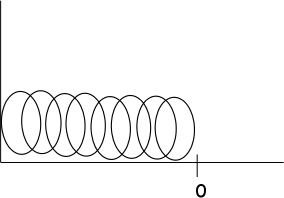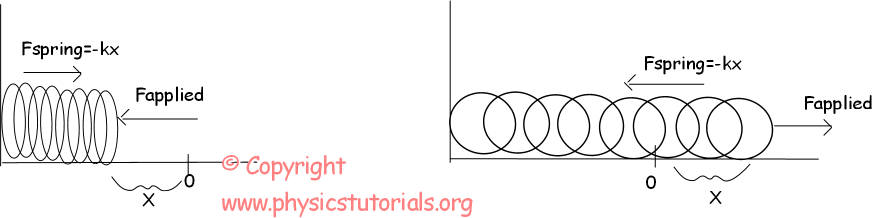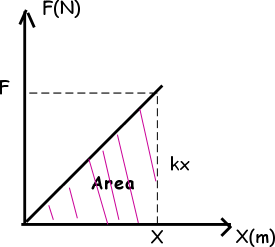# Potential Energy with Examples

Article Index
Potential Energy
Potential Energy Continue
All Pages

POTENTIAL ENERGY OF THE SPRING

By compressing the spring or stretching it you load a potential energy to it. Well, if I apply same force to different springs having different thicknesses, are they loaded with the same energy? I hear that you all say no! You are absolutely right. Of course the thinner spring is more compressed than the thicker one where the quantity of compression shows the loaded potential energy. What I want to say is that, potential energy of the spring depends on the type of spring and the amount of compression. The mathematical representation of this definition is given below.

Ep=1/2.k.x²

Where k is the spring constant and x is the amount of compression.Now, we look at the origin of this formula. This is a picture of a spring at rest. There is no compression or stretching. Thus, we can not talk about the potential energy of the spring. However, in the pictures given below springs are not at rest position. Let’s examine the behavior of the springs in two situations.In the first picture, we apply a force, Fapplied, and spring reacts this force with Fspring=-kx. The amount of compression is X. In the second picture we stretch the spring by the amount of X. We apply force of F and spring gives reaction to this force with Fspring=-kx where x is the stretching amount and k is the spring constant. The given graph below is force versus distance graph of springs. We find the energy equation of spring by using this graph.

As I said before area under the force vs distance graph gives us the work and energy is the capability of doing work. So, area under this graph must give us the potential energy of the spring.

Area=1/2.F.x=1/2.kx.x=1/2kx²

Ep=1/2kx²

Example 50N of force is applied to a spring having 150N/m spring constant. Find the amount of compression of the spring.Fspring=-kx=Fapplied

50N=-150.x

X=-3m “-“shows the direction of compression.

Work Power Energy Exams and Solutions

Related